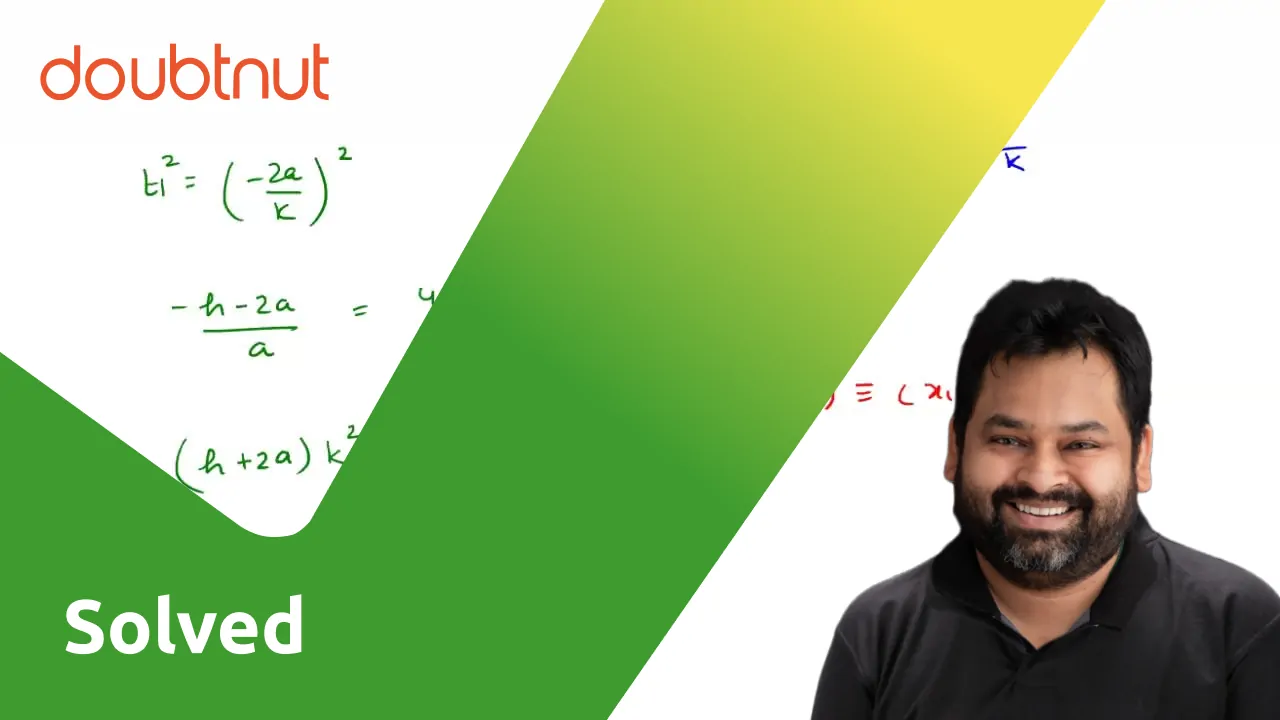Doubtnut App - Get 50 Lakh+ video solutions better than GoogleInstall Now

# Tangents are drawn at the end points of a normal chord of the parabola y2=4ax. The locus of their point of intersection is

A

(x2a)y2+4a3=0

B

(x2a)y24a3=0

C

(x+2a)y24a3=0

D

(x+2a)y2+4a3=0

Text Solution
Verified by Experts

Video Solution